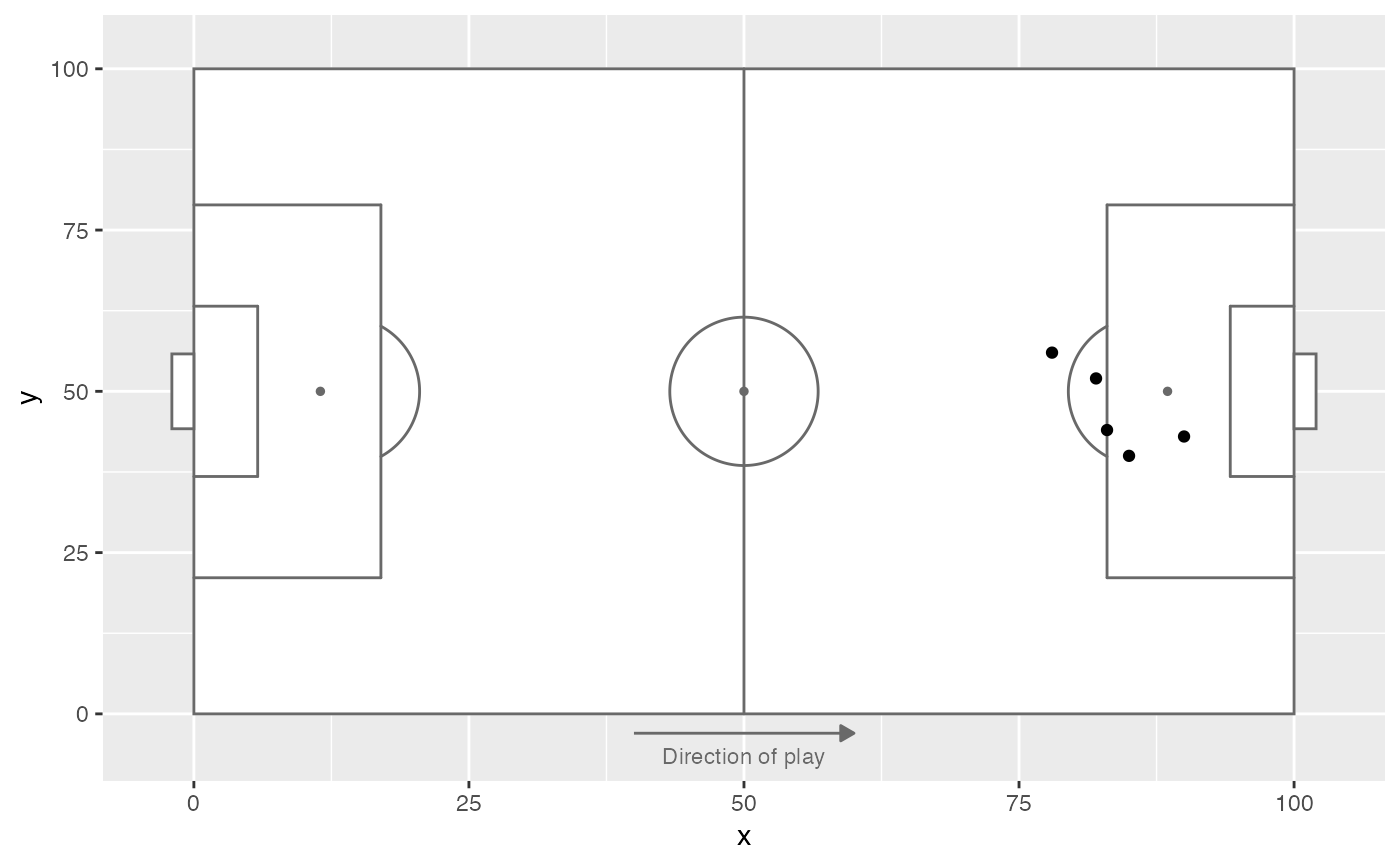Adds an arrow indicating the direction of play to a ggplot plot

direction_label(
x_label = 50,
y_label = -3,
label_length = 20,
colour = "dimgray"
)

Arguments

x_label

x position of the centre of the arrow on the plot

y_label

y position of the arrow on the plot

label_length

length of arrow (in x axis units)

colour

colour of the arrow and text

Value

list of ggplot layers to be added to a ggplot plot

Examples

shots_data <- data.frame(x = c(90, 85, 82, 78, 83),
y = c(43, 40, 52, 56, 44))

p <- ggplot(shots_data, aes(x = x, y = y)) +

# Add direction of play label
p + direction_label()